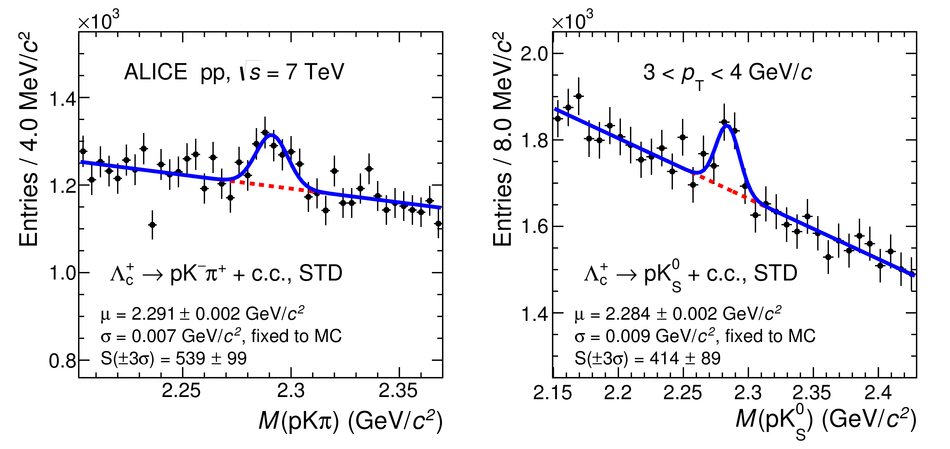# Figure 3

 Invariant-mass distribution of $\Lcplus$ candidates (and charge conjugates) for $3 <$ \pt $< 4$ \gevc in pp collisions at \sqrts = 7~\tev. The dashed lines represent the fit to the background while the solid lines represent the total fit function. Left: $\LctopKpi$ STD analysis, right: $\LctopKzS$ STD analysis.